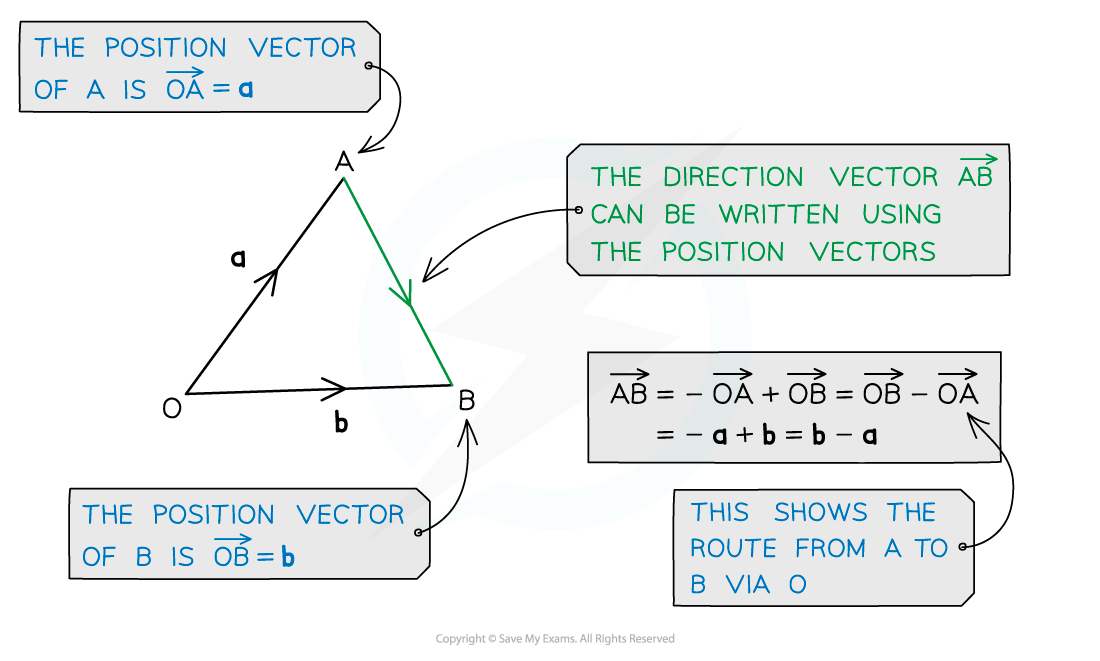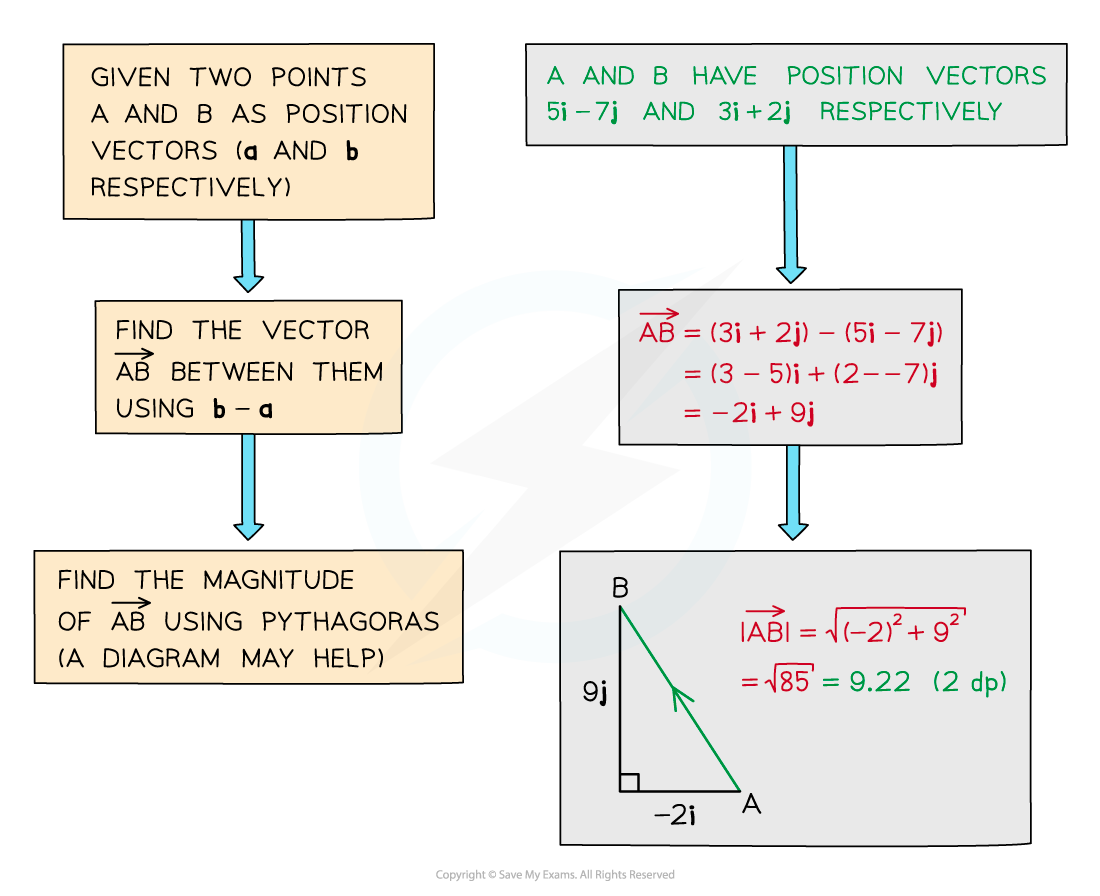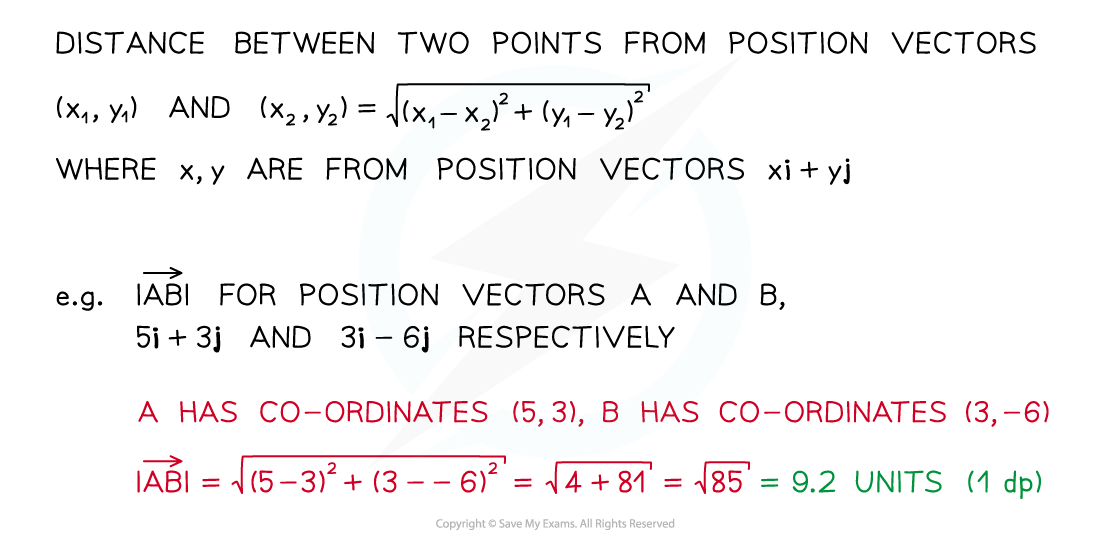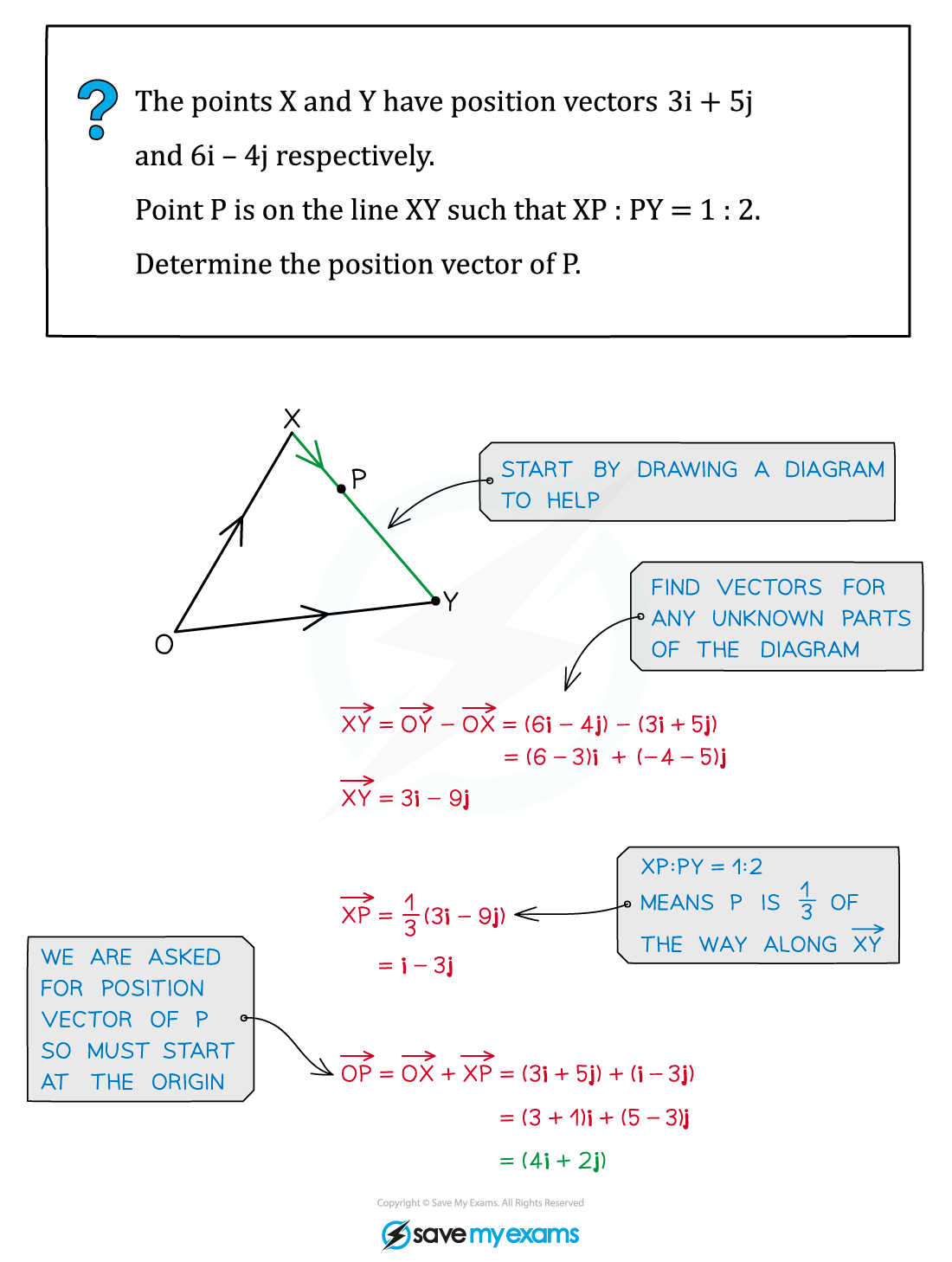# CIE A Level Maths: Pure 3复习笔记7.1.4 Position Vectors

### Position Vectors

#### What is a position vector?

• Position vectors describe the position of a point in relation to the origin
• They are different to direction vectors which describe the direction and distance between any two points#### Distance between two points

• The distance between two points is the magnitude of the vector between them (see Magnitude Direction)• For position vectors we can find the distance between two points by using the respective co-ordinates#### Exam Tip

• Remember if asked for a position vector, you must find the vector all the way from the origin.
• Diagrams can help, if there isn’t one, draw one.

#### Worked Example